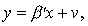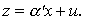Multiprocess
Multilevel ModelingaML

product info
multilevel example
multiprocess example

### A Multiprocess Example

While there are several software packages on the market which support multilevel modeling, none offers aML's capabilities to mix outcome types in multiprocess (multi-equation) settings. Consider a simple Heckman selection model. The continuous outcome of interest is modeled by a linear model:but it is only observed if some z>0, whereFor example, wages y are only observed for people who work (z>0). If people with poor wage prospects disproportionately decide not to work, u and v are correlated. In that case, the first equation may not be estimated independently from the second. In aML, you may specify the model as follows:

```define regressor set AlphaX;
var = <list of variables>;
define regressor set BetaX;
var = <list of variables>;

define normal distribution; dim=2;
name=u;
name=v;

probit model;
outcome = z;
model = regressor set AlphaX +
residual(draw=1, ref=u);

continuous model; keep if (z>0);
outcome = y;
model = regressor set BetaX +
residual(draw=1, ref=v);
```
The model equations are specified in an intuitive manner following their mathematical representations. Residuals u and v are correlated across equations because they are defined as part of the same distribution and have the same "draw."

The example readily generalizes to other types of outcomes and to multilevel models. For example, you may estimate Heckman-type probit selection models ("heckprob" models), multilevel Heckman selection models, and further embed such models into a larger system of equations. Similarly, multivariate heterogeneity may be correlated across equations.